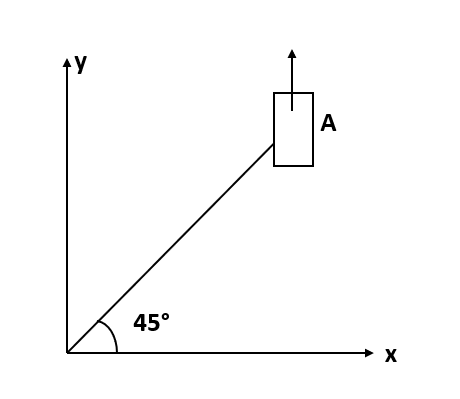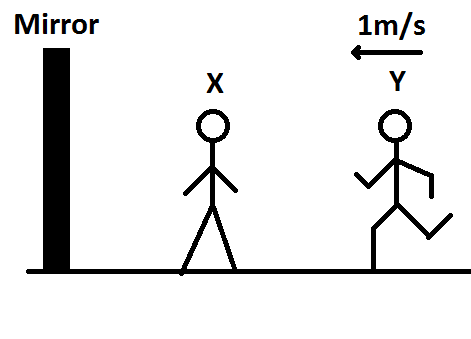Filters
Latest Questions
CBSE
Physics
Relative motion in one dimension
A body is thrown up in a lift with a velocity $u$ relative to the lift and the time of flight is $t$ . Show that the lift is moving up with an acceleration $\dfrac{{2u - tg}}{t}$.
CBSE
Physics
Relative motion in one dimension
Two trains travelling on the same track are approaching each other with equal speed of $40\,m\,{s^{ - 1}}$ . The drivers of the trains begin to decelerate simultaneously when just $2.0\,km$ apart. Assuming the decelerations to be uniform and equal, the value to the deceleration to barely avoid collision should be
A. $11.8\,m\,{s^{ - 2}}$
B. $11.0\,m\,{s^{ - 2}}$
C. $2.1\,m\,{s^{ - 2}}$
D. $0.8\,m\,{s^{ - 2}}$
CBSE
Physics
Relative motion in one dimension
A train $110\,m$ in length travels at $60$ per hour. In what time it will pass a man who is walking at $6\,km$ an hour in the opposite direction.
A. $5\sec$
B. $6\sec$
C. $8\sec$
D. $7\dfrac{1}{3}\sec$
CBSE
Physics
Relative motion in one dimension
Two parallel rail tracks run north-south. On one track train A moves north with a speed of 54 km/h and on the other track train B moves south with a speed of 90 km/h. The velocity of train A with respect to train B is:
A. 10m/s
B. 15m/s
C. 25m/s
D. 40m/s
CBSE
Physics
Relative motion in one dimension
A large wooden raft is flowing with a river stream. A man on the raft dives into the river and swims down the stream for 15 minutes and then he turns about and comes back to the raft. The swimming speed of man is 5km/h in still water and the speed of the river is 4km/h. The distance moved by the raft till the man comes back to raft is
A. 1 km
B. 2 km
C. 3 km
D. 4 km
CBSE
Physics
Relative motion in one dimension
A body leaving a certain point O moves with an acceleration which is constant. At the end of the first second after it left O, its velocity is $1.5 ms^{-1}$. At the end of the sixth second after it left O the body stops momentarily and begins to move backwards. The distance travelled by the body before it stops momentarily is
a) $9 m$
b) $18 m$
c) $5.4 m$
d) $36 m$
CBSE
Physics
Relative motion in one dimension
On a frictionless horizontal surface, assumed to be the x-y plane., a small trolley A is moving along a straight line parallel to the y-axis with a constant velocity of $(\sqrt{3} -1 ) ms^{-1}$. At a particular instant, when the OA line makes an angle of $45^{\circ}$ with the axis, a ball is thrown along the surface from the origin O. Its velocity makes an angle $\phi$ with the x-axis and it hits the trolley.a. The motion of the ball is observed from the frame of the trolley. Calculate the angle $\theta$ made by the velocity vector of the ball with the x-axis in this frame.
b. Find the speed of the ball with respect to the surface, if $\phi = \dfrac{4 \theta}{3}$ .
CBSE
Physics
Relative motion in one dimension
A man can swim at the rate of $5Km/hr$in still water. A$1Km$wide river flows at the rate of $3km/hr$.The man wishes to swim across the river directly opposite to the starting point.
a. Along what direction must the man swim?
b. What should be his resultant velocity?
c. How much time will he take to cross the river?
CBSE
Physics
Relative motion in one dimension
A ball is dropped from a building of height 45 m. Simultaneously another identical ball B is thrown up with a speed $50\,m{{s}^{-1}}$. The relative speed of ball B w.r.t ball A at any instant of time is (Take $g=10\,m{{s}^{-2}}$).
\begin{align} & A.\,0 \\ & B.\,10\,m{{s}^{-1}} \\ & C.\,25\,m{{s}^{-1}} \\ & D.\,50\,m{{s}^{-1}} \\ \end{align}
CBSE
Physics
Relative motion in one dimension
Man X is standing in front of the plane mirror while man Y is running towards him from behind. If the man Y is running at a speed of $1m\;{s^{ - 1}}$ , how many metres nearer does the manY seem to be away from man X after $5seconds$ ?(A) $7m$
(B) $5m$
(C) $6m$
(D) $10m$

IIT-JEE
Physics
Relative motion in one dimension
A motor boat going downstream overcame a raft at a point $A$. $60\min$ later it turns back & after some time it passes a raft again at a distance $6km$ from point $A$.Find the flow of velocity assuming the speed of the boat is constant.
IIT-JEE
Physics
Relative motion in one dimension
A person walks up a stalled escalator in 90 seconds. When standing on the same escalator, now moving, he is carried in 60 second. The time it would take him to walk up the moving escalator will be:
A) 27 s
B) 72 s
C) 18 s
D) 36 s
Prev
1
2
3
4
5